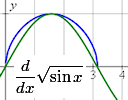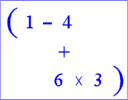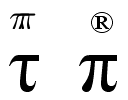Archive for March, 2011

IntMath Newsletter: Free resources, derivative from first principles

1. Free resource: Study Tips from Cornell University
2. Have your say - how should math education be changed?
3. Free resource: Space Math @ NASA
4. Derivative of square root of sine x by first principles
5. GeoGebra is part of Google Summer of Code 2011
6. Lesson idea for order of operations
7. Final thought – enthusiasm

Derivative of square root of sine x by first principlesHere's how to find the derivative of √(sin x) from first principles.

Lesson idea for order of operationsHere's a motivating way for students to learn order of arithmetic operations.

GeoGebra is part of Google Summer of Code 2011This is an interesting summer project - write some math-related software for Google!

IntMath Newsletter: Pi Day, new obesity index, resources

14 Mar 2011 - Pi Day

1. Latest feedback - IntMath reduces math anxiety
2. Let's drop pi
3. New measure of obesity - body adiposity index (BAI)
4. Resource - FREE (Federal Resources for Educational Excellence)
5. Friday math movies: (a) Pi Day Song and (b) Conrad Wolfram on Teaching kids real math with computers
6. Final thought – Positive results

Let’s drop piShould we use a symbol other than pi? Is pi wrong?

Friday math movie: Pi Day SongHere's a fun song about learning the digits of pi - and some of its characteristics.

New measure of obesity - body adiposity index (BAI)Researchers have suggested a new way to determine body fat - the BAI. What is the math behind it?

Friday math movie: Conrad Wolfram on Teaching kids real math with computersMathematics is boring when it concentrates on calculation rather than understanding and application. Conrad Wolfram, one of the developers of Wolfram|Alpha, shares his views.

Full archive

See the blog sitemap for all previous articles.